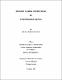## Second layer conditions in noetherian ringsThe hierarchy of the various versions of the second layer condition, transferability of the second layer condition to extension rings, and relativization of it with respect to a torsion theory are investigated. We show that the strong second layer condition is indeed stronger than the ordinary second layer condition by introducing an intermediate condition, called the right restricted strong second layer condition, and its equivalent versions in Chapter 2. The second layer condition is passed to certain extension rings. Letzter proved that if R is a noetherian ring satisfying the second layer condition and S is an overring of R such that $\sb{R}S$ and $S\sb{R}$ are finitely generated, then S satisfies the second layer condition. In Chapter 3, we e tend this result to a noetherian ring satisfying just the right second layer condition. The proof makes use of finite annihilation of tame modules, thus dispensing with a symmetric dimension function implicitly required in Letzter's proof. It is not known if the polynomial ring $R\lbrack x\rbrack$ satisfies the right second layer condition when R is a right noetherian ring with right second layer condition. We show that $R\lbrack x\rbrack$ satisfies the right second layer condition when R is a noetherian very strongly right AR-separated ring. Also, we observe that if E$(R\lbrack x\rbrack /P)\sb{R\lbrack x\rbrack }$ is $(P \cap R)$-tame for each prime P of $R\lbrack x\rbrack$, then $R\lbrack x\rbrack$ satisfies the right second layer condition. Let $\sigma$ be a torsion radical on Mod-R, where R is a right $\sigma$-noetherian ring. In Chapter 4, we show that R is right fully $\sigma$-bounded iff Spec$\sb\sigma(R)$ satisfies the right restricted strong second layer condition and R has local bijective Gabriel correspondence. Prior to this, we establish that the local bijective Gabriel correspondence with respect to $\sigma$ is equivalent to $\sigma$-torsionfree modules being tame. We also investigate localization of R with respect to a perfect torsion radical $\sigma$ and finite annihilation of E$(R/P)$, where P is a minimal prime ideal of R.